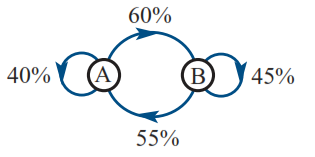6152 Setting Up Transition Matrices

8 Questions | Total Attempts: 115Settings• 1.
Create a transition matrix, T, to represent the network diagram above. Use columns to define the starting point.  What is the value of ?
• 2.
Create a transition matrix, T, to represent the network diagram above. Use columns to define the starting point.  What is the value of  ?
• 3.
Create a transition matrix, T, to represent the network diagram above. Use columns to define the starting point.  What is the value of  ?
• 4.
Create a transition matrix, T, to represent the network diagram above. Use columns to define the starting point.  What is the value of  ?
• 5.
Create a transition matrix, T, to represent the network diagram above. Use columns to define the starting point.  What is the value of  ?
• 6.
Create a transition matrix, T, to represent the network diagram above. Use columns to define the starting point.  What is the value of  ?
• 7.
Create a transition matrix, T, to represent the network diagram above. Use columns to define the starting point.  What is the value of  ?
• 8.
Create a transition matrix, T, to represent the network diagram above. Use columns to define the starting point.  What is the value of  ?
• 9.
Create a transition matrix, T, to represent the network diagram above. Use columns to define the starting point.  What is the value of  ?
• 10.
Create a transition matrix, T, to represent the network diagram above. Use columns to define the starting point.  What is the value of  ?
• 11.
Create a transition matrix, T, to represent the network diagram above. Use columns to define the starting point.  What is the value of  ?
• 12.